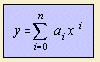# polynomial

## mainA polynomial is literally 1) an expression of some terms, distinct powers in one ore more variables. On this page we talk about a polynomial in one variable, x. When talking about two variables (x and y), we call it an algebraic curve. A curve for which f(x,y) is a constant, is called a equipotential curve. Other names for the curve are: isarithm, isopleth. As said, here the polynomial in x, each term is an entire power of x, and the function is also called an entire (rational) function. It is also called an algebraic function. The highest power in x is the degree (or: order) of the polynomial. An entire function of 2nd degree is called a quadratic function; an entire function of 4th degree is called an biquadratic function. Some specific polynomials can be found as: line y is linear in x. parabola y is quadratic in x. cubic quartic The root to the nth power 1/n is the inverse function of the (simple) polynomial with equation y = xn. A broken function is a function that is the quotient of two polynomials.Together with the polynomials do they form the group of rational functions, also named the rational polynomial functions. It was Pierre de Fermat who found the curves with equation y = xn, therefore: for positive n the curve is a parabola of Fermat for negative n the curve is a hyperbola of Fermat The following polynomials have a special significance: notes 1) polus (Gr.) = many, nomen (Lat.)  = name.